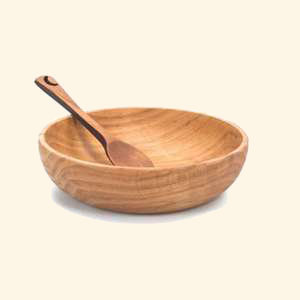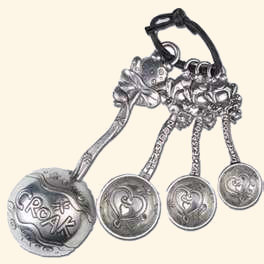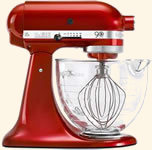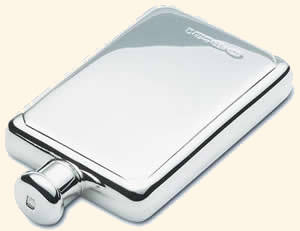Cooking Conversions# Imperial Fluid Ounces to Millilitres Conversions (fl oz to ml)

For smaller amounts of liquid in your recipe you will often see fluid ounces (fl oz) or millilitres (ml) as the units. This page gives the conversions from Imperial fluid ounces to millilites and millilitres to Imperial fluid ounces, for US recipes, where the measurements are in US fluid ounces you will need the US fluid ounces to millilitres conversion.

For larger liquid measures we also have Imperial pints to litres conversion and Imperial pints to US pints conversion

## Imperial Fluid Ounces to Millilitres Converter

Type a value in either field to convert from Imperial fluid ounces to millilitres (UK fl oz to ml) or millilitres to Imperial fluid ounces(ml to UK fl oz) (results are accurate to 2 decimal places).

 UK Fluid oz Millilitres UK fl oz = ml

## Common Imperial Fluid Ounce to Millilitre Conversion Table

Conversions for Imperial fluid ounce to millilitres values often useed in recipes are given in the table below (results are rounded to the nearest millitre).

 Imperial Fluid Ounces ¼ ½ ¾ 1 1½ 2 3 4 6 8 10 12 15 16 20 Millilitres 7 14 21 28 43 57 85 114 170 227 284 341 426 455 568

## Impreial Fluid Ounces to Millilitres Conversion Formula

The conversion formula for Imperial fluid ounces to millilitres (UK fl oz to ml) is 1 Imperial fluid ounce = 28.4130625 millilitres.

## Common Millilitre to Imperial Fluid Ounce Conversion Table

Values commonly used in cooking for millilitre to Imperial fluid ounce conversions are given in the table below (results are rounded to 2 decimal places).

 Millilitres 1 5 10 15 20 25 30 40 50 100 250 500 1000 Imperial Fluid Ounces 0.04 0.18 0.35 0.53 0.7 0.88 1.06 1.41 1.76 3.52 8.8 17.6 35.2

## Millilitre to Imperial Fluid Ounce Conversion Formula

The conversion formula for millilitres to Imperial fluid ounces (ml to UK fl oz) is 1 millilitre = 0.0351951 Imperial fluid ounces.

Other liquid measure conversions for cooking